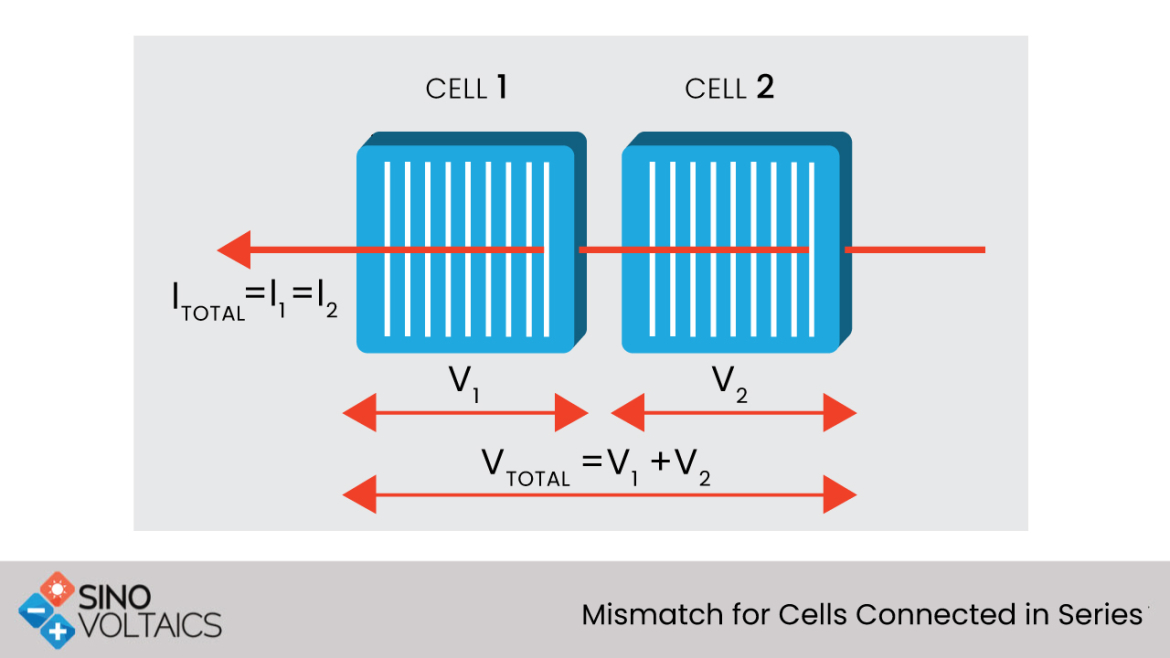# Cells Connected in Series

### Mismatch for Cells Connected in series

As the majority of PV modules are connected in series, series mismatches are the most common type of mismatch faced. Between the two simplest types of mismatch considered (short circuit current mismatch or in open circuit voltage mismatch), the short circuit current mismatch is more common, as it can be easily caused by the shading of a part of the module. This type of mismatch is the most severe type as well.For any two cells connected in series, the current through the two cells is equal. The total voltage produced is the sum of the voltages of the individual cells. Since the produced current must be equal, any mismatch in current means that the total current produced from the configuration is equal to the lowest current.

#### Open Circuit Voltage Mismatch for Series connected cells

A mismatch in the open circuit voltage of cells connected in series is a relatively simple form of mismatch. At short circuit current, the overall current produced from the PV module remains unaffected. At the Mpp (maximum power point), the overall power is decreased as the solar cell is generating less power. Since the two cells are connected in series, the current through both solar cells is equal, and the overall voltage is determined by adding the two voltages at a specific current.#### Short Circuit Current Mismatch for Series connected cells

The mismatch in the short circuit current of solar cells connected in series can, based on the operating point of the module and the degree of mismatch, have a severe impact on the PV module. At open circuit voltage, the effect of a reduced short circuit current is relatively minor. There is a minimum change in the open circuit voltage due to the logarithmic dependence of open circuit voltage on the short circuit current. Yet, since the current across the two cells must be equal, the total current from the combination cannot exceed that of the lower or mismatched cell. Thus, the current from the configuration cannot exceed the short circuit current of the lower cell.At lower voltages where this condition is expected to occur, the extra current generating capability of the cells is not dissipated in each individual cell (as normally occurring at short circuit), but instead is dissipated in the mismatched or poor cell.Overall, in a configuration connected in series that has a current mismatch, severe power reductions are experienced if the mismatched cell produces less current than the maximum power current of the proper cells and also if the combination is operated at short circuit or at low voltages, the dissipation of high power in the poor cell can cause an irreversible damage to the module.By

M.VISHNU

on 01 Oct 2022

I have a doubt, Question is-In the solar panel the cells are connected in series to increased the voltage .In the series connection the current(amps) is constant but in solar panel, why the current is increasing according to the temperature.

Place comment